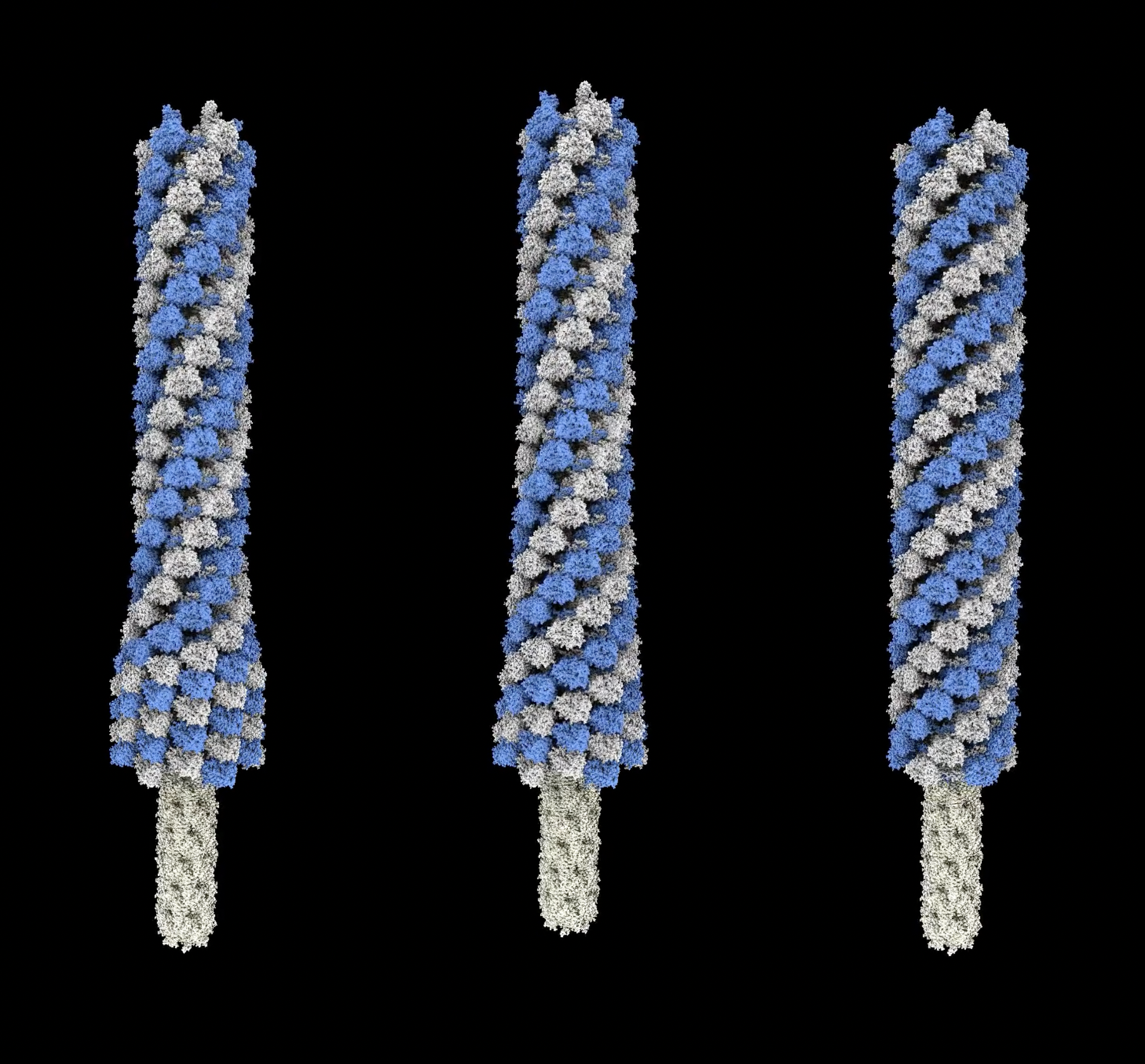# Morph a phage helical tube

Here we morph a helical tube made of proteins from one pitch to another starting at one end and progressing to the other end in a wave. This is a model of how phage tails contract to push a needle through a target cell through which the phage injects its genome. The wave propagation is described in

```    Quantitative description of a contractile macromolecular machine
Alec Fraser, Nikolai S Prokhorov, Fang Jiao, B Montgomery Pettitt, Simon Scheuring, Petr G Leiman
```

and we animate it for the phage T4 tail described in

```    Cryo-EM Structure and Assembly of an Extracellular Contractile Injection System
Jiang F, Li N , Wang X, Cheng J, Huang Y, Yang Y, Yang J, Cai B, Wang YP, Jin Q, Gao N
Cell, 2019, 177, 370-383
```

We morph the extended helix (rise 39.3 Angstroms, twist 19.9 degrees) to the contracted helix (rise 17.0 Angstroms, twist 31.4 degrees) and the protein conformation from the extended structure PDB 6j0b to the contracted conformation PDB 6j0c.

First I align a single 6-protein ring of the extended and contracted helices, center at the origin, adjust chain identifiers so they match, morph the conformation, and save this single ring morph as 6j0c_6j0b_morph.pdb as shown in ChimeraX command script helixalign.cxc. The script also saves the core needle tube needle.cif which is inside the contracting helix and does not change. Then I use the Python helixwave.py code that defines the helixwave command to animate the wave transition from extended to contracted helix.

``````open helixalign.cxc
close
open needle.cif
color #1 lightyellow
open 6j0b_6j0c_morph.pdb coordset true
color #2 lightgray
color #2/A,C,E cornflowerblue
light soft
open helixwave.py
helixwave #2 28 move #1
``````

To record a movie

``````movie record ; helixwave #3 move #1 ; wait 300 ; movie encode helixwave.mp4
``````

or using a smaller contraction zone (0.2 times full length)

``````movie record ; helixwave #3 wavelength 0.2 move #1 ; wait 300 ; movie encode helixwave_0.2.mp4
``````

or uniform contraction zone

``````movie record ; helixwave #3 uniform true move #1 ; wait 300 ; movie encode helixwave_uniform.mp4
``````

Here is a movie of the 0.2 wavelength, 0.5 wavelength and uniform motion side by side. This can be used in an animation including the phage capsid and baseplate.Here is the Python code helixwave.py

```    #
# Animate Phage T4 sheath contraction as a wave starting at the tip and progressing to the capsid.
# Use PDB 3j0b extended (rise 17A, twist 31.4 degrees) and 3j0c (rise 39.3A, twist 19.9 degrees),
# 28 layers.
#
# We animate each of the 28 layers using a rotation and translation about the sheath axis,
# and a conformation change of the monomers from extended to contracted.  The first layer is at
# z = 0 and layers are stacked in z.  The last layer is the tip and does not rotate or
# translate.  The first layer (where the virus capsid is attached) rotates and moves along z
# and additional models such as a capsid and needle model can be moved with it using the
# move_models parameter.
#
# This animation is a bit tricky because rotating and translating one layer also rotates and
# translates all the layers at lower z.
#

def helix_wave(session, layer_models, num_layers = 28, time = None, steps = 300,
wave_length = 0.5, uniform = False,
rise_start = 39.3, rise_end = 17.0, twist_start = 19.9, twist_end = 31.4,
move_models = None):
'''
If a single model is specified then num_layers copies will be made, otherwise
the specified models will be treated as the layers.
The layer model should be a morph of a single layer between the two helix states
centered at the origin (0,0,0) with tube axis along z.
'''
if len(layer_models) == 1:
layer_model = layer_models
layer_models = [layer_model.copy() for l in range(num_layers)]
layer_model.display = False

if time is None:
def wave(session, frame):
time = frame/(steps-1)
show_wave(layer_models, time, wave_length, uniform,
rise_start, rise_end, twist_start, twist_end, move_models)
from chimerax.core.commands.motion import CallForNFrames
CallForNFrames(wave, steps, layer_models.session)
else:
show_wave(layer_models, time, wave_length, uniform,
rise_start, rise_end, twist_start, twist_end, move_models)

def show_wave(layer_models, time, wave_length, uniform,
rise_start, rise_end, twist_start, twist_end, move_models = None):
ztrans = zrot = 0
profile = wave_profile(time, len(layer_models), wave_length, uniform)
for layer_model, f in zip(layer_models, profile):
ztrans += (1-f) * rise_start + f * rise_end
zrot += (1-f) * twist_start + f * twist_end
from chimerax.geometry import translation, rotation
layer_model.position = translation((0,0,ztrans)) * rotation((0,0,1), zrot)
coordset_ids = layer_model.coordset_ids
layer_model.active_coordset_id = coordset_ids[int(f * (len(coordset_ids)-1))]

# Keep the helix tip fixed
nlayer = len(layer_models)
height, twist = (nlayer-1)*rise_start, (nlayer-1)*twist_start
start_tip_position = translation((0,0,height)) *  rotation((0,0,1), twist)
r = start_tip_position * layer_models[-1].position.inverse()
for m in layer_models:
m.position = r * m.position

# Move models as if fixed to the helix base.
if move_models is not None:
r = layer_models.position
for m in move_models:
if not hasattr(m, '_wave_start_positions'):
m._wave_start_positions = m.positions
m.positions = r * m._wave_start_positions

def wave_profile(time, num_layers, wave_length, uniform):
'''
Return fraction contracted for each layer at a given time.  Last layer contracts first.
Time ranges from 0 to 1, fully extended to fully contracted.
'''
if uniform:
profile = [time]*num_layers
else:
l0 = (1 - time * (1 + wave_length))*(num_layers-1)
l1 = l0 + wave_length * num_layers
profile = []
for layer in range(num_layers):
if layer <= l0:
f = 0
elif layer >= l1:
f = 1
else:
f = (layer-l0)/(l1-l0)
profile.append(f)
return profile

def register_command(session):
from chimerax.core.commands import CmdDesc, register, FloatArg, IntArg, BoolArg
from chimerax.atomic import AtomicStructuresArg
desc = CmdDesc(required=[('layer_models', AtomicStructuresArg)],
optional=[('num_layers', IntArg)],
keyword=[('time', FloatArg),
('steps', IntArg),
('wave_length', FloatArg),
('uniform', BoolArg),
('rise_start', FloatArg),
('rise_end', FloatArg),
('twist_start', FloatArg),
('twist_end', FloatArg),
('move_models', AtomicStructuresArg),
],
synopsis='Morph a twist and contract wave along a helical filament')
register('helixwave', desc, helix_wave, logger=session.logger)

register_command(session)
```

Tom Goddard, September 11, 2022